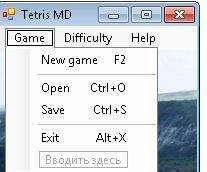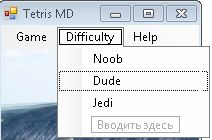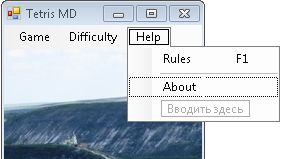# Creating Tetris Using Visual C++ Windows Form. Part two.

In the first article I described the process of creating a `Shape` class that encapsulates the logic of the Tetris game. No we will create an form, that will present the interface of the Tetris game. The first thing is to create the form appearance. I set a background image in the properties tab in Visual Studio. After this I added a menu strip to have 3 menu items: 1.2.3.All these things can be done in Visual Studio Form constructor by simply dragging elements from element's panel. Now we need to interact with the created `Shape` class to play a Tetris game. The form class needs to have these member variable:
`Shape^ shape;    array<Int16,2>^ stock;//game board    Point^ curPoint;//curent point    Boolean	bGame;    Boolean _pause;    Boolean firstView;//used to show welcome screen    Int16	score;    Double	level;    Int16 color; // current color    System::Timers::Timer^ t1;`
The constructor of the class initializes these variables:
`Form1(void)    {        stock = gcnew array<Int16,2>(20,10);        shape = gcnew Shape();	_pause  = false;	firstView = true;	Invalidate();         SetLevel(1);        InitializeComponent();        //Don'tchange window size        MaximizeBox     = false;     }`
The all drawing of the program is done in onPaint function. As you can see in the code above, there is a variable, called `firstView`. It's used in the onPaint function to show welcome screen:
`if(firstView){System::Drawing::Font^ drawFont = gcnew System::Drawing::Font( "Arial",16 );SolidBrush^ drawBrush = gcnew SolidBrush( Color::Black );PointF drawPoint = PointF(10.0F,50.0F);grfx->DrawString("Tetris.\nChose difficulty\n and press \nF2 to play",drawFont, drawBrush,drawPoint);firstView = false;}`
The next step is to set event handlers for all menu items for the game menu. A double click on a menu item in constructor creates a handler for this item. The new menu item click starts new game:
`System::Void newGameToolStripMenuItem_Click(System::Object^  sender, System::EventArgs^  e)    {	firstView = false;	SetLevel(1);	this->t1 = gcnew System::Timers::Timer(level);	this->t1->Elapsed += gcnew System::Timers::ElapsedEventHandler(this, &Form1::TimerEventProcessor);        ClearStock();        score=0;        bGame=true;        NewShape();        this->Invalidate();    }`
A timer is started in this function. It's done to change the position of the figure from top to bottom, according to the selected level. The event handler of the timer changes the position of the shape and checks if the game is not over of a line is completed:
` if(bGame==true)        {            if(IsAtBottom())            {                for(Int16 i=0; i<4; i++)                    for(Int16 j=0; j<4; j++)                    {                        if(shape->cells[i,j]==true)                            stock[curPoint->Y/20+i,curPoint->X/20+j]=color;                    }                NewShape();                if(IsAtBottom())                {                    bGame=false;                }                this->Invalidate();                CheckForLine();                return;            }            curPoint->Y+=20;            this->Invalidate();        }        else if(bGame==false)        {            this->t1->Stop();            MessageBox::Show("You lost !\rYour score is : "+score);        }`
The new shape function produces a new shape of the random type:
`void NewShape(void) // Novaia figura    {        t1->Start();        curPoint = gcnew Point(0,60);        Random^   rand = gcnew Random();        ShapeType t = ShapeType(rand->Next(7));        shape->NewShape(t);        color=rand->Next(6);    };`
This function changes the position of the shape to the initial. Reset's its color and type. The event handler for pressed key checks the possibility to move shape to left or right, pause and restart the game,rotates the shape and invalidates the region,where the shape is located:
` System::Void Form1_KeyDown(System::Object^  sender, System::Windows::Forms::KeyEventArgs^  e)    {        switch(e->KeyCode)        {        case Keys::Up :			if(IsAtBottom()||CheckRight()) return;			shape->Rotate();			this->Invalidate(GetReg());            break;        case Keys::Left:            if(CheckLeft()) return;            curPoint->X-=20;			this->Invalidate(GetReg());            break;        case Keys::Right:            if(CheckRight()) return;            curPoint->X+=20;			this->Invalidate(GetReg());            break;        case Keys::Down:            while(!IsAtBottom()){				curPoint->Y+=20;				this->Invalidate(GetReg());			}             break;		case Keys::Pause:            if(!_pause){				t1->Stop();				_pause = true;			}			else{				t1->Start();				_pause = false;			}			this->Invalidate();            break;        default :            break;        }    }`
The checks of the possibilities to move are performed by the following functions thanks to the coordinated of the current position of the shape:
` Boolean CheckLeft(void) // vojmojnosti idti nalevo    {        for(Int16 i=0; i!=4; i++)        {            for(Int16 j=0; j!=4; j++)            {                if(shape->cells[i,j]==true)                {                    if((curPoint->X/20+j)<=0 || stock[curPoint->Y/20+i,curPoint->X/20+j-1]!=-1)                        return true;                }            }        }        return false;    }    Boolean CheckRight(void) // vojmojnosti dvigatsia vpravo    {        for(Int16 i=0; i != 4; i++)        {            for(Int16 j=0; j!=4; j++)            {                if(shape->cells[i,j]==true)                {                    if((curPoint->X/20+j)>=9 || stock[curPoint->Y/20+i,curPoint->X/20+j+1]!=-1)                        return true;                }            }        }        return false;    } `
We need to add points and clear a line when is filled. This check is done here in the following way:
`void CheckForLine(void)    {        Int16 i,j,k,c=0;        Boolean flag;         for(i=0; i<20; i++)        {            flag=true;            for(j=0; j<10; j++)                flag &= (stock[i,j]!=-1);             if(flag==true && j!=0)            {                c++;                score++;                 for(k=i; k>0; k--)                {                    for(j=0; j<10; j++)                    {                        stock[k,j]=stock[k-1,j];                    }                }            }        }        for(k=0; k<c; k++)            for(j=0; j<10; j++)                stock[k,j]=-1;        this->Invalidate();    }`
And the last check is to check, if the Shape is in the bottom:
`    Boolean IsAtBottom(void)     {        for(Int16 i=0; i<4; i++)        {            for(Int16 j=0; j<4; j++)            {                if(shape->cells[i,j]==true)                {                    if((curPoint->Y/20+i)>=19 || stock[curPoint->Y/20+i+1,curPoint->X/20+j]!=-1)                        return true;                }            }        }        return false;    }`
The OnPaint function simply draws the rectangles of different colors according to the values, that are in stock:
`Graphics^ grfx = pea->Graphics;        Int16 i,j;        SolidBrush^ brush = gcnew SolidBrush(ShapeColor(color));         for(i=0; i<4; i++)        {            for(j=0; j<4; j++)            {                if(shape->cells[i,j]==true)                    grfx->FillRectangle(brush,curPoint->X+j*size, curPoint->Y+i*size,size,size);            }        }         for(i=0; i<20; i++)        {            for(j=0; j<10; j++)            {                if(stock[i,j]!=-1)                {                    brush->Color =  ShapeColor(stock[i,j]);                    grfx->FillRectangle(brush,j*size,i*size,size,size);                }            }        } 		if(_pause)		{			 System::Drawing::Font^ drawFont = gcnew System::Drawing::Font( "Arial",20 );			SolidBrush^ drawBrush = gcnew SolidBrush( Color::Black );			PointF drawPoint = PointF(10.0F,150.0F);    		 grfx->DrawString("Game paused.\nPress \"Pause\"\n to continue",drawFont, drawBrush,drawPoint);		}`
Here are the complete details about how to implement the Tetris user interface as a Visual c++ Windows Forms Application.You can download the complete source code of this Visual Studio 2010 Project.